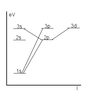# The bohr model - dusting off

• liquidFuzz
In summary, the first levels of an atom are composed of electrons with different numbers. The number of electrons is based on the quantum number n. The rules for determining the quantum number are based on the principle quantum number n and the azimuthal quantum number l. The number of electrons in a state is written in superscript and the principle quantum number is also written in superscript. The rules for writing quantum states use the principle quantum number and the name of a sub-shell that contains the orbitals. The number of electrons in a state is also written in superscript. The rules for writing quantum states also use the principle quantum number and the name of a sub-shell that contains the orbitals. The number of electrons inf

#### liquidFuzz

I'm sitting with Physics Handbook and I'm trying to recall how the make out the orbitals in the energy levels. Let's take a look at the first levels.

n = 1 - sub-shell K
Number of electrons = $2n^2 \Rightarrow 2$ electrons.
Number of electrons = $l = 0,1... n-1 \Rightarrow l = 0$.

This is where I get stuck. I can't remember how to get the orbitals; s, p, d etc...

Edit, is n = 0 & l = 0 written as 1s?

Last edited:
Ah well, I think I've got it, using brute force on my F.H. One thing though.

I seem to remember using this model to decide between which orbitals a jump is possible. Is it, how would I do that if yes..?

Cheers!

I'm not sure of the terminology in English. Would this be called Electron Transition? The rules, how do I determine $\Delta m_l$ ? I added a picture of what I've got so far.

Rules

$\Delta l = \pm 1$
$\Delta m_l = 0, \pm 1$

#### Attachments

•hydrogen.gif
3.5 KB · Views: 470
First write down the principle quantum no. n the azimuthal quantum number is l=n-1 and then magnetic quantum number is m=-l to l. n and l give energy states, and m gives orientation of degenerate states. n can never be zero here at in the which you are dealing with. n=1 l=0 is written as 1s.
for s, l=0
for p, l=1
for d, l=2
for f, l=3
for g, l=4 and so on..
Actually s, p, d, f, ... are initial alphabets of the spectral terms - sharp,principle,diffuse,fundamental.
general rule for writing quantum states is -(principle quntum no. n)(name of sub-shell s,p,d or f)and number of electron in that state in superscript.
One more thing s, p, d, f,... are subshells containing orbitals decided by m and each orbital contains at max. 2 electrons as per Pauli's exclusion principle.
s-> one orbital m=0,
p-> three degenerate orbitals m=-1,0,1
and so on

Thanks for the explanation. I tried to sketch possible transitions... Is any transitions but 1s --> 2p and 1s --> 3p likely to occur given that they have to stop at 2p?